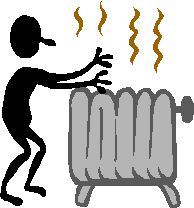# Unit 2: Energy Rules!

### Section A. Introduction

This unit will look at how energy can be transferred and converted from one form to another, how energy efficiency is define and measured, and the natural laws that limit how much useful energy can be obtianed by a process.

## Energy Transfer

Energy can be transferred from one object or system to another in two ways:1. Doing work, such as pushing an object2. Transferring heat through conduction, convection, and radiation

## Energy Conversion and the First Law of Thermodynamics

Energy can neither be created nor destroyed, it can only be converted from one form to another. This is the first law of thermodynamics.

For example, the chemical energy in coal can be converted into thermal energy. Every unit of chemical energy is converted into the same number of units of thermal energy, all of which can be accounted for without any energy being created out of nothing or vanishing into nothing.

## Energy Efficiency and the Second Law of Thermodynamics

The energy efficiency of a conversion is the ratio of the output of useful energy with respect to the original energy. No conversion process is 100 percent energy efficient. This is because every unit of energy before a conversion cannot be converted into the same number of useful units of energy after a conversion, because some of the original energy is dispersed into the environment and is unavailable for doing anything useful afterward. This is the second law of thermodynamics.

For example, the thermal energy released by burning coal is eventually dispersed into the environment. This dispersed energy has a lower "energy quality" in that it is difficult to put it to any use. The measure of this lower energy quality due to being dispersed is called "entropy." The entropy of an unburned piece of coal and its surroundings is lower than the entropy of the ashes, cinders, and the warmed surroundings due to burning that piece of coal.​ ​​ Continue on to Section B: Energy Transfer